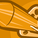# QlikView App Dev

Discussion Board for collaboration related to QlikView App Development.

Announcements
Join this live chat April 6, 10AM EST - QlikView to Qlik Sense REGISTER
cancel
Showing results for
Did you mean:Contributor II

## filter pivot table with original column

Hello community,

In a pivot table i'm trying to build an expression, in this expression I want to sum column A values when column B is equal to X. column B is also a pivoting dimension on the same table so I've tried with:

=sum( if(columnB=X, Alt(columnA,0) ))

or

=sum( {1<columnB= {X}>} Alt(columnA,0) )

but the expression only consider the values of columnB of that part of the chart.

I would like to consider all the data of the original table ignoring the current chart position.

I'm trying to be as clear possible but I'm still learning basic qlik's terminology.

Also I'm not sure about the place to post this in

1 Solution

Accepted SolutionsContributor II
Author

I found out that the TOTAL keyword was what I was searching so my solution is:

=sum( TOTAL if(columnB=X, Alt(columnA,0) ))

4 RepliesSpecialist

Hi Ricardo

Would you be able to share a sample?MVP

May be this?

=sum( {1<columnB *= {X}>} Alt(columnA,0) )

Before develop something, think If placed (The Right information | To the right people | At the Right time | In the Right place | With the Right context)Contributor II
Author

I found out that the TOTAL keyword was what I was searching so my solution is:

=sum( TOTAL if(columnB=X, Alt(columnA,0) ))MVP